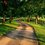# [Differential Geometry] On the Smooth Equivalence of Parametrised Differentiable Curves.

We say that two regular parametric curves $$\phi$$ and $$\psi$$ are smoothly equivalent, if there is a strictly monotonically increasing differentiable function $$s$$ such that $$\psi(s(t)) = \phi(t)$$. Reparameterisation means that you have parametrised $$\psi$$ by some new parameter $$s(t)$$ that is a function of $$t$$, instead of the original parameter, $$t$$. For example, you can reparameterise a curve with its arc length, given as a function of $$t$$.

Why does $s$ have to be monotonically increasing rather than decreasing? $s$ must be monotonically increasing, because we want to preserve the orientation (i.e. direction through which it travels) of the curve.

Are the follwing two curves $\phi(\theta) = (cos(\theta),sin(\theta)),$

$\psi(\theta) = (cos(2\theta),sin(2\theta)),$

smoothly equivalent if the domain of $\phi$ is $[0,2\pi]$, the domain of $\psi$ is $[0,\pi]$?

These two curves have the same trace, but are not considered to be equivalent curves. The curves have distinct velocities, which you can easily see when you calculate tangent vectors at each of the points on the curves, $\psi'(\theta)=2\cdot\phi'(\theta),$

so $\psi(\theta)$ travels twice as fast as $\phi(\theta)$. Note that this means that you cannot distinguish parametrised differentiable curve simply by looking at their image sets. Smoothly equivalent parametrised curves can be reparametrised from one to the other, and thus we obtain an equivalence class of regular curves.

Below is a list of parametrised differentiable curves that all trace out a unit circle in $\mathbb{R}^2$.

$\phi_1(t) = \cos t + i\sin t, ~ t \in \left[0 \leq t \leq 2\pi \right],$

$\phi_2(t) = \cos(2\pi t^{2}) + i\sin(2\pi t^{2}),~ t \in \left[ 0 \leq t \leq 1 \right],$

$\phi_3(t) = \cos t + i\sin t, ~t \in \left[ 0 \leq t \leq 4\pi \right],$

$\phi_4(t) = \cos (-t) + i\sin(-t), ~t \in \left[ 0 \leq t \leq 2\pi \right].$

Note that $\phi_1(t)$ and $\phi_2(t)$ are mutually smoothly equivalent, because they are reparametrisations of each other traced in the same direction. However, $\phi_3(t)$ traces the unit circle twice while $\phi_1(t)$ traces it once, so they are not smoothly equivalent. Also, $\phi_4(t)$ traces the unit circle clockwise, while $\phi_1(t)$ traces it anticlockwise, so they are not smoothly equivalent.Note by Bright Glow
2 years, 11 months ago

This discussion board is a place to discuss our Daily Challenges and the math and science related to those challenges. Explanations are more than just a solution — they should explain the steps and thinking strategies that you used to obtain the solution. Comments should further the discussion of math and science.

When posting on Brilliant:

• Use the emojis to react to an explanation, whether you're congratulating a job well done , or just really confused .
• Ask specific questions about the challenge or the steps in somebody's explanation. Well-posed questions can add a lot to the discussion, but posting "I don't understand!" doesn't help anyone.
• Try to contribute something new to the discussion, whether it is an extension, generalization or other idea related to the challenge.
• Stay on topic — we're all here to learn more about math and science, not to hear about your favorite get-rich-quick scheme or current world events.

MarkdownAppears as
*italics* or _italics_ italics
**bold** or __bold__ bold
- bulleted- list
• bulleted
• list
1. numbered2. list
1. numbered
2. list
Note: you must add a full line of space before and after lists for them to show up correctly
paragraph 1paragraph 2

paragraph 1

paragraph 2

[example link](https://brilliant.org)example link
> This is a quote
This is a quote
    # I indented these lines
# 4 spaces, and now they show
# up as a code block.

print "hello world"
# I indented these lines
# 4 spaces, and now they show
# up as a code block.

print "hello world"
MathAppears as
Remember to wrap math in $$ ... $$ or $ ... $ to ensure proper formatting.
2 \times 3 $2 \times 3$
2^{34} $2^{34}$
a_{i-1} $a_{i-1}$
\frac{2}{3} $\frac{2}{3}$
\sqrt{2} $\sqrt{2}$
\sum_{i=1}^3 $\sum_{i=1}^3$
\sin \theta $\sin \theta$
\boxed{123} $\boxed{123}$

## Comments

There are no comments in this discussion.

×

Problem Loading...

Note Loading...

Set Loading...